# Fredholm theorems

(diff) ← Older revision | Latest revision (diff) | Newer revision → (diff)

for integral equations

## Contents

### Theorem 1.

The homogeneous equation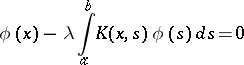(1)

and its transposed equation(2)

have, for a fixed value of the parameter, either only the trivial solution, or have the same finite number of linearly independent solutions: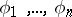;.

### Theorem 2.

For a solution of the inhomogeneous equation(3)

to exist it is necessary and sufficient that its right-hand side be orthogonal to a complete system of linearly independent solutions of the corresponding homogeneous transposed equation (2):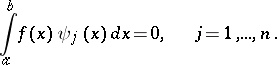(4)

### Theorem 3.

(the Fredholm alternative). Either the inhomogeneous equation (3) has a solution, whatever its right-hand side, or the corresponding homogeneous equation (1) has non-trivial solutions.

### Theorem 4.

The set of characteristic numbers of equation (1) is at most countable, with a single possible limit point at infinity.

For the Fredholm theorems to hold in the function space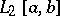it is sufficient that the kernelof equation (3) be square-integrable on the set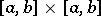(andmay be infinite). When this condition is violated, (3) may turn out to be a non-Fredholm integral equation. When the parameterand the functions involved in (3) take complex values, then instead of the transposed equation (2) one often considers the adjoint equation to (1):In this case condition (4) is replaced by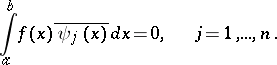These theorems were proved by E.I. Fredholm .